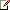# Double.Equals Method (Object)

Microsoft Silverlight will reach end of support after October 2021. Learn more.

Returns a value indicating whether this instance is equal to a specified object.

Namespace:  System
Assembly:  mscorlib (in mscorlib.dll)

## Syntax

``````'Declaration
Public Overrides Function Equals ( _
obj As Object _
) As Boolean
``````
``````public override bool Equals(
Object obj
)
``````

#### Parameters

• obj
Type: System.Object
An object to compare with this instance.

#### Return Value

Type: System.Boolean
true if obj is an instance of Double and equals the value of this instance; otherwise, false.

## Remarks

The Equals method should be used with caution, because two apparently equivalent values can be unequal due to the differing precision of the two values. The following example reports that the Double value .3333 and the Double returned by dividing 1 by 3 are unequal.

``````' Initialize two doubles with apparently identical values
Dim double1 As Double = 0.33333
Dim double2 As Object = 1 / 3
' Compare them for equality
outputBlock.Text &= double1.Equals(double2) & vbCrLf    ' displays False
``````
``````// Initialize two doubles with apparently identical values
double double1 = .33333;
object double2 = 1 / 3;
// Compare them for equality
outputBlock.Text += double1.Equals(double2) + "\n";    // displays false
``````

Rather than comparing for equality, one recommended technique involves defining an acceptable margin of difference between two values (such as .01% of one of the values). If the absolute value of the difference between the two values is less than or equal to that margin, the difference is likely to be due to differences in precision and, therefore, the values are likely to be equal. The following example uses this technique to compare .33333 and 1/3, the two Double values that the previous example found to be unequal.

``````' Initialize two doubles with apparently identical values
Dim double1 As Double = 0.33333
Dim double2 As Object = 1 / 3
' Define the tolerance for variation in their values
Dim difference As Double = Math.Abs(double1 * 0.0001)

' Compare the values
' The output indicates that the two values are equal
If Math.Abs(double1 - CDbl(double2)) <= difference Then
outputBlock.Text &= "double1 and double2 are equal." & vbCrLf
Else
outputBlock.Text &= "double1 and double2 are unequal." & vbCrLf
End If
``````
``````// Initialize two doubles with apparently identical values
double double1 = .33333;
object double2 = (double)1 / 3;
// Define the tolerance for variation in their values
double difference = Math.Abs(double1 * .0001);

// Compare the values
// The output indicates that the two values are equal
if (Math.Abs(double1 - (double)double2) <= difference)
outputBlock.Text += "double1 and double2 are equal." + "\n";
else
outputBlock.Text += "double1 and double2 are unequal." + "\n";
``````

In this case, the values are equal.Note:

Because Epsilon defines the minimum expression of a positive value whose range is near zero, the margin of difference between two similar values must be greater than Epsilon. Typically, it is many times greater than Epsilon.

The precision of floating-point numbers beyond the documented precision is specific to the implementation and version of the .NET Framework. Consequently, a comparison of two particular numbers might change between versions of the .NET Framework because the precision of the numbers' internal representation might change.

## Examples

The following example demonstrates the Equals method.

``````Obj1 = CType(500, Double)

If A.Equals(Obj1) Then
outputBlock.Text &= "The value type and reference type values are equal." & vbCrLf
End If
``````
``````obj1 = (Double)500;
if (a.Equals(obj1))
{
outputBlock.Text += "The value type and reference type values are equal." + "\n";
}
``````

## Version Information

#### Silverlight

Supported in: 5, 4, 3

#### Silverlight for Windows Phone

Supported in: Windows Phone OS 7.1, Windows Phone OS 7.0

#### XNA Framework

Supported in: Xbox 360, Windows Phone OS 7.0

## Platforms

For a list of the operating systems and browsers that are supported by Silverlight, see Supported Operating Systems and Browsers.